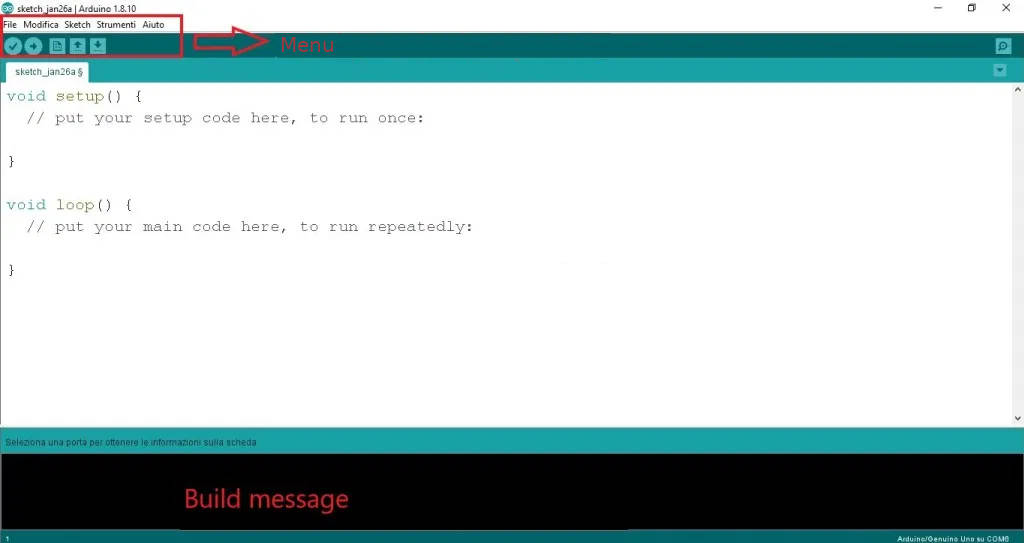# Arduino IDE: arithmetic and logical operators #3Let’s start defining the environment needed to improve our programming skills on Arduino.

In the previous article of this guide we learnt how to assign a value to a variable. A variable can be assigned a data or the result of an expression. An expression is a formula that always specifies a value (or result). Each expression is made up of operators and operands.

Operands can be constants, expressions or variables.

Operators can be of three types: arithmetic, relationship and logical.

The arithmetic operators are:

• – for subtraction
• * for multiplication
• / for the division
• % to calculate the remainder of the division between integers

Arithmetic operators perform the classic operations between two numbers such as sum and difference.

Examples:

Relationship (or comparison) operators are used to compare the contents of two variables and are indicated with symbols:

• == equal to (comparison between two variables)
• <less than
• <= less than or equal to
• > greater
• > = greater than or equal to
• ! = different

The relationship (or comparison) operators are used in conditional and iterative statements (if, while, do … while, etc.). If the condition is verified, it returns true, otherwise false. The relationship (or comparison) operators usually need two arguments and are positioned between them.

So we could write:

In the first case, I assign a value of 5 to a, while in the second case I compare a with 5 and it returns the Boolean value true if a equals 5, otherwise it returns false.

So let’s make some examples:

• (a > b) – returns true if condition a greater than b has occurred, false otherwise
• (a >= b) – returns true if condition a greater than or equal to b has occurred, false otherwise
• (a < b) – returns true if the condition a less than b is verified, false otherwise
• (a <= b) – returns true if condition a less than or equal to b has occurred, false otherwise
• (a == b) – returns true if condition a equal to b has occurred, false otherwise
• (a != b) – returns true if condition a other than b is verified, false otherwise

So for example if a = 5 and b = 7 then the expressions will be true: (a <b), (a <= b) and (a! = b).

While if for example a = 7 and b = 7 the expressions that will be true will be: (a <= b), (a >= b) and (a == b).

The logical operators are:

• && indicates the conjunction operation (and)
• || indicates the disjunction operation (or)
• ! indicates negation (not)How do these logical connectives work?

AND: If both operators are true then the output is true; in all other cases the output is equal to

 A B A and B V V V V F F F V F F F F

OR: If at least one of the operands is equal to true, the output is true; otherwise, if neither operand is equal to true the output will be false.

 A B A or B V V V V F V F V V F F F

NOT: If the input operand is true then the output will be false. If, on the other hand, the input operand is false, then the output will be equal to true. In other words, the NOT operator takes an input and returns the exact opposite.

 A not A V F F V

The use of operators is fundamental in drafting a code and in solving a problem.

Previous articles:

Follow us to keep yourself updated with the news!

### Simone CandidoSimone Candido è un ragazzo appassionato del mondo tech nella sua totalità. Simone ama immedesimarsi in nuove esperienze, la sua filosofia si basa sulla irrefrenabile voglia di ampliare a 360° le sue conoscenze abbracciando tutti i campi del sapere, in quanto ritiene che il sapere umano sia il connubio perfetto tra cultura umanistica e scientifica.

This site uses Akismet to reduce spam. Learn how your comment data is processed.